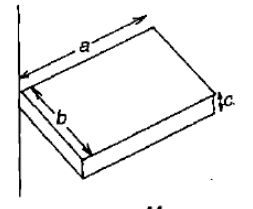A solid cylinder and a hollow cylinder, both of the same mass and same external diameter are released from the same height at the same time on an inclined plane. Both roll down without slipping. Which one will reach the bottom first?

1.  Both together only when the angle of inclination of the plane is ${45}^{0}$

2.  Both together

3.  Hollow cylinder

4.  Solid cylinder

High Yielding Test Series + Question Bank - NEET 2020

Difficulty Level:

The moment of inertia of a rod about an axis through its centre and  perpendicular to it is $\frac{1}{12}M{L}^{2}$ (where M is the mass and L is the length of the rod). The rod is bent in the middle so that the two halves make an angle of 60$°$. The moment of inertia of the bent rod about the same axis would be

(1) $\frac{1}{48}M{L}^{2}$

(2) $\frac{1}{12}M{L}^{2}$

(3) $\frac{1}{24}M{L}^{2}$

(4) $\frac{M{L}^{2}}{8\sqrt{3}}$

Concept Questions :-

Moment of inertia
High Yielding Test Series + Question Bank - NEET 2020

Difficulty Level:

A man is sitting on a rotating table with his arms stretched outwards.  When he suddenly folds his arms inside, then

(1) his angular velocity will decrease

(2) his angular velocity remains constant

(3) his moment of inertia decreases

(4) angular momentum increases

High Yielding Test Series + Question Bank - NEET 2020

Difficulty Level:

A body of mass M and radius R is rolling horizontally without slipping with speed v.  It then rolls up a hill to a maximum height h.  If $h=\frac{5{v}^{2}}{6g}$, what is the M.I of the body?

(1) $\frac{M{R}^{2}}{2}$                   (2)

(3)                 (4)

High Yielding Test Series + Question Bank - NEET 2020

Difficulty Level:

One projectile moving with velocity v in space, gets burst into 2 parts of masses in the ratio 1:3.  The smaller part becomes stationary.  What is the velocity of the other part?

(1) 4v                        (2) v

(3) $\frac{4v}{3}$                     (4) $\frac{3v}{4}$

High Yielding Test Series + Question Bank - NEET 2020

Difficulty Level:

One circular ring and one circular disc both having the same mass and radius. The ratio of their moments of inertia about the axes passing through their centres and perpendicular to planes will be

1. 1:1

2. 2:1

3. 1:2

4. 4:1

Concept Questions :-

Moment of inertia
High Yielding Test Series + Question Bank - NEET 2020

Difficulty Level:

A wheel of radius R rolls on the ground with a uniform velocity v. The velocity of topmost point relative to the bottommost point is

1. v

2. 2v

3. v/2

4. zero

Concept Questions :-

Rolling motion
High Yielding Test Series + Question Bank - NEET 2020

Difficulty Level:

The figure shows a uniform solid block of mass M and edge lengths a, b and c. Its M.O.I. about an axis through one edge and perpendicular (as shown) to the large face of the block is1. $\frac{M}{3}\left({a}^{2}+{b}^{2}\right)$

2. $\frac{M}{4}\left({a}^{2}+{b}^{2}\right)$

3. $\frac{7M}{12}\left({a}^{2}+{b}^{2}\right)$

4. $\frac{M}{12}\left({a}^{2}+{b}^{2}\right)$

Concept Questions :-

Moment of inertia
High Yielding Test Series + Question Bank - NEET 2020

Difficulty Level:

The moment of inertia of a cube of mass m and side a about one of its edges is equal to

(1)                 (2)

(3)                   (4)

Concept Questions :-

Moment of inertia
High Yielding Test Series + Question Bank - NEET 2020

Difficulty Level:

If the net external forces acting on the system of particles is zero, then which of the following may vary ?

1. Momentum of the system

2. Velocity of centre of mass

3. Position of centre of mass

4. None of the above

Concept Questions :-

Center of mass